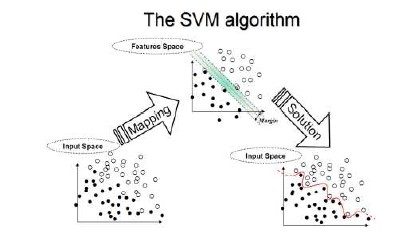﻿ how to train SVM using MATLAB?Back

## how to train SVM using MATLAB?SVM - Support vector machine with MATLAB

I encountered that very few students actually know how to do image processing and most of all there is no place to find a good tutorial for beginners who do not want to go by theoretical knowledge and would want to get their hands dirty with MATLAB programming. So, my blog is targeted for those students who wants to work in this field and unfortunately are not able to find any relevant information on machine learning algorithms and programming with MATLab anywhere on Internet.

Image can be processed in plenty of ways and one of them which I will present to you is on machine learning algorithms which I will be using on MATLAB. I will keep my language as basic as possible for beginners to understand, no offense to professionals as we all were in a learning phase in our life.

My tutorial will follow a very basic structure as follows:

Obtaining the Image Datasets - ( I will be using Caltech101 dataset )

Separate Training set and Test set images.

Creating Lables for SVM train to distinct class.

Training SVM

Classify Test set images.

At the moment, I will assume that you are familiar with the term machine learning algorithms. I have absolutely zero intention to discuss theory over here.

Just for beginners,
Training set - This set of images will be used to train our SVM.
Test set - In the end of the svm training we will use these images for classification.
Label - I will use Faces and Airplanes, these are two objects so we will give them two "labels".
Classify - Distinguish our test set images.

Finally, I will present you a simple code for classification using SVM. I have used the Caltech101 dataset for this experiment. Train dataset will consist of 30 images divided in two class and two labels will be provided to them. Code is very basic to be understood. Hope it helps. The program goes as follows:

Prepatory steps:
Training set - Create a folder with 15 "Faces" images and 15 "airplanes" images, this will be our dataset.
Test set - Create another folder with random face and airplanes images, this will be our testset, basically we have to understand here is that if you use training set images as test set images then you will get 100% recognition performance.
--------------------------------------------------------------------------------------------------------
clc
clear all

Dataset = 'absolute path of the folder';
Testset = 'absolute path of the folder';

% we need to process the images first.
% Convert your images into grayscale
% Resize the images

width=100; height=100;
DataSet = cell([], 1);

for i=1:length(dir(fullfile(Dataset,'*.jpg')))

% Training set process
k = dir(fullfile(Dataset,'*.jpg'));
k = {k(~[k.isdir]).name};
for j=1:length(k)
imgInfo = imfinfo(horzcat(Dataset,filesep,k{j}));

% Image transformation
if strcmp(imgInfo.ColorType,'grayscale')
DataSet{j} = double(imresize(tempImage,[width height])); % array of images
else
DataSet{j} = double(imresize(rgb2gray(tempImage),[width height])); % array of images
end
end
end
TestSet = cell([], 1);
for i=1:length(dir(fullfile(Testset,'*.jpg')))

% Training set process
k = dir(fullfile(Testset,'*.jpg'));
k = {k(~[k.isdir]).name};
for j=1:length(k)
imgInfo = imfinfo(horzcat(Testset,filesep,k{j}));

% Image transformation
if strcmp(imgInfo.ColorType,'grayscale')
TestSet{j} = double(imresize(tempImage,[width height])); % array of images
else
TestSet{j} = double(imresize(rgb2gray(tempImage),[width height])); % array of images
end
end
end

% Prepare class label for first run of svm
% I have arranged labels 1 & 2 as per my convenience.
% It is always better to label your images numerically
% Please note that for every image in our Dataset we need to provide one label.
% we have 30 images and we divided it into two label groups here.
train_label = zeros(size(30,1),1);
train_label(1:15,1) = 1; % 1 = Airplanes
train_label(16:30,1) = 2; % 2 = Faces

% Prepare numeric matrix for svmtrain
Training_Set=[];
for i=1:length(DataSet)
Training_Set_tmp = reshape(DataSet{i},1, 100*100);
Training_Set=[Training_Set;Training_Set_tmp];
end

Test_Set=[];
for j=1:length(TestSet)
Test_set_tmp = reshape(TestSet{j},1, 100*100);
Test_Set=[Test_Set;Test_set_tmp];
end

% Perform first run of svm
SVMStruct = svmtrain(Training_Set , train_label, 'kernel_function', 'linear');
Group = svmclassify(SVMStruct, Test_Set);

------------------------------------------------------------------------------------------------------------

Finally, you can check you Image recognition performance by seeing Group variable. you can also try to give the same dataset and testset location and you will achieve 100% recognition. This is because the same image is being classified which you are using to train you svm.